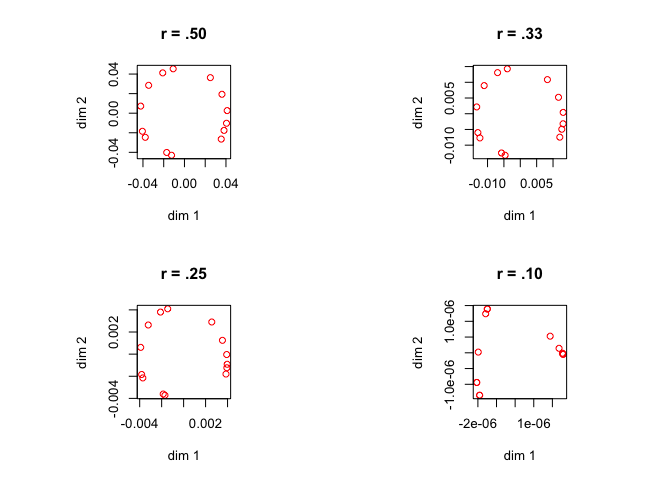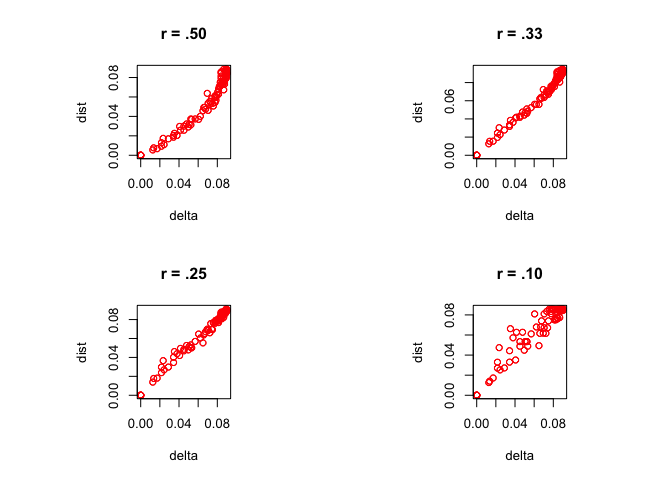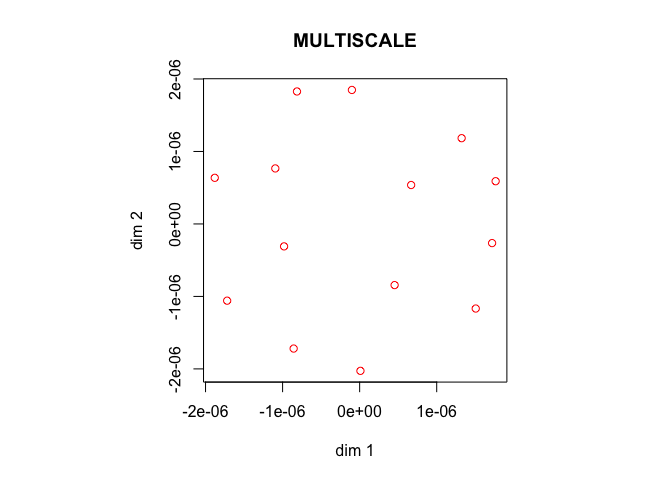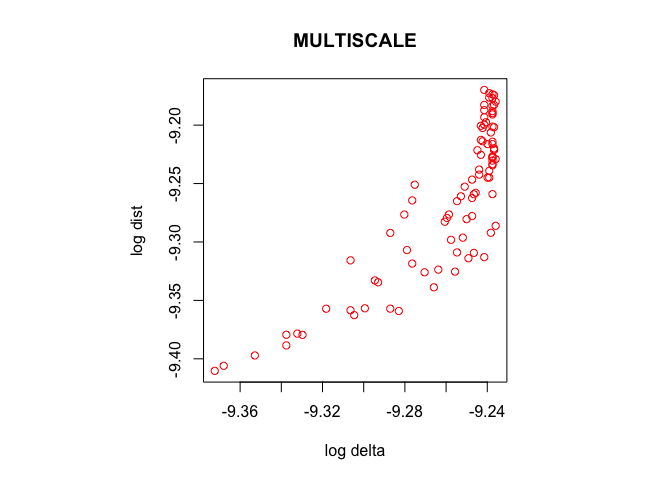Note: This is a working paper which will be expanded/updated frequently. All suggestions for improvement are welcome. The directory deleeuwpdx.net/pubfolders/qstress has a pdf version, the complete Rmd file with all code chunks, the bib file, and the R source code.

# 1 Introduction

We define qStress as $\begin{equation} \sigma_q(x):=\sum_{i=1}^n w_i (\delta_i-(x'A_ix)^q)^2,\label{E:qs} \end{equation}$

where the $$\delta_i$$ are dissimilarities between pairs of objects and the $$d_i(x)=x'A_ix$$ are squared Euclidean distances between pairs of points in $$\mathbb{R}^p$$.

The properties of qStress, and algorithms to minimize it for various values of q, have been discussed in Groenen and De Leeuw (2010), De Leeuw (2014), De Leeuw, Groenen, and Mair (2016b), De Leeuw, Groenen, and Mair (2016a), De Leeuw, Groenen, and Mair (2016c). In this paper we develop a new majorization algorithm for $$0<q\leq\frac12$$, based on an elementary inequality for power funcions.

Without loss of generality we assume $\sum_{i=1}^n w_i\delta_i^2=1.$ We also expand the sum of squares to decompose qStress into two additive components that can be analyzed, and majorized, separately. The notation is the same as that used by De Leeuw (1977). $\begin{equation} \sigma_q(x)=1-2\rho_q(x)+\eta_q(x),\label{E:qstress} \end{equation}$ where \begin{align} \eta_q(x)&:=\sum_{i=1}^n w_i(x'A_ix)^{2q},\label{E:eta}\\ \rho_q(x)&:=\sum_{i=1}^n w_i\delta_i(x'A_ix)^{q}.\label{E:rho} \end{align}

# 2 Preliminaries

The basis of our treatment is a family of simple inequalities for powers of non-negative numbers.

Lemma 1: [Power]

1. For $$z>0$$ and $$r\leq 0$$ we have $$z^r\geq rz + (1-r)$$.
2. For $$z>0$$ and $$0\leq r\leq 1$$ we have $$z^r\leq rz + (1-r)$$.
3. For $$z>0$$ and $$r\geq 1$$ we have $$z^r\geq rz + (1-r)$$.

In all three cases we have equality if and only if $$z=1$$.

Proof: The derivative of $$f(z)=z^r-rz$$ vanishes at $$z=1$$, and $$f(1)=1-r$$. Because $$f''(1)=r(r-1)$$ we see that $$f$$ has a minimum at 1 if $$r<0$$ or $$r>1$$ and it has a maximum at 1 if $$0<r<1$$. QED

Equivalently, the inequalities follow from the convexity of $$z^r$$ for $$r\geq 1$$ or $$r\leq 0$$ and the concavity of $$z^r$$ for $$0\leq r\leq 1$$.

We can apply lemma 1 to powers of ratios of quadratic forms.

Lemma 2: [Forms] Suppose $$A$$ is positive definite, $$x$$ and $$y$$ are arbitrary vectors, $$s$$ and $$t$$ are arbitrary real numbers. Then $\begin{equation} (x'Ax)^{sr+t}\leq r(x'Ax)^{s+t}(y'Ay)^{s(r-1)}+(1-r)(x'Ax)^t(y'Ay)^{sr}\label{E:leq} \end{equation}$ for $$0\leq r\leq 1$$ and $\begin{equation} (x'Ax)^{sr+t}\geq r(x'Ax)^{s+t}(y'Ay)^{s(r-1)}+(1-r)(x'Ax)^t(y'Ay)^{sr}.\label{E:geq} \end{equation}$

for $$r\geq 1$$ and $$r\leq 0$$. There is equality if and only if $$x'Ax=y'Ay$$.

Proof: From lemma 1 $\left[\left(\frac{x'Ax}{y'Ay}\right)^s\right]^r\lessgtr r\left(\frac{x'Ax}{y'Ay}\right)^s+(1-r),$ with $$>$$ or $$<$$ depending on $$r$$. Thus $(x'Ax)^{rs}\lessgtr r(x'Ax)^s(y'Ay)^{s(r-1)}+(1-r)(y'Ay)^{rs},$ and multiplying both sides by $$(x'Ax)^t$$ gives the result. QED

# 3 qStress

In order to majorize $$\sigma_q$$ we first minorize $$\rho_q$$ and then majorize $$\eta_q$$.

Lemma 3: [Rho] If $$A$$ is positive definite, $$x$$ and $$y$$ are arbitrary, and $$q\leq\frac12$$, then $(x'Ax)^q\geq (2q-1)(x'Ax)(y'Ay)^{q-1}+2(1-q)(x'Ax)^\frac12(y'Ay)^{q-\frac12},$ with equality if and only if $$x'Ax=y'Ay$$.

Proof: Take $$s=t=\frac12$$ and $$r=2q-1$$ in equation $$\eqref{E:geq}$$ in lemma 2. QED

Lemma 4: [CS] If $$A$$ is positive definite, $$x$$ and $$y$$ are arbitrary, and $$q\leq\frac12$$, then $(x'Ax)^q\geq (2q-1)(x'Ax)(y'Ay)^{q-1}+2(1-q)(x'Ay)(y'Ay)^{q-1},$ with equality if and only if $$x'Ax=y'Ay$$.

Proof: By Cauchy-Schwartz $$(x'Ax)^\frac12\geq(y'Ay)^{-\frac12}(x'Ay)$$. Substitute this in lemma 3. QED

Lemma 5: [Eta] If $$A$$ is positive definite, $$x$$ and $$y$$ are arbitrary, and $$0\leq q\leq\frac12$$, $(x'Ax)^{2q}\leq 2q(x'Ax)(y'Ay)^{2q-1}+(1-2q)(y'Ay)^{2q},$ with equality if and only if $$x'Ax=y'Ay$$.

Proof: Take $$s=1$$ and $$t=0$$ and $$r=2q$$ in equation $$\eqref{E:leq}$$ in lemma 2. QED

For the next theorem, our main majorization result, we define

\begin{align} S(y)&:=\sum_{i=1}^n w_i(y'A_iy)^{2q-1}A_i,\label{E:Sy}\\ T(y)&:=\sum_{i=1}^n w_i\delta_i(y'A_iy)^{q-1}A_i.\label{E:Ty} \end{align} Theorem 1: [Majorize] For $$0\leq q\leq\frac12$$ $\sigma_q(x)\leq 1+\gamma(y)+x'V(y)x-2x'B(y)y,$ where \begin{align} V(y)&:=2\{qS(y)-(2q-1)T(y)\},\label{E:V}\\ B(y)&:=2(1-q)T(y),\label{E:B} \end{align} and $\begin{equation} \gamma(y):=(1-2q)\sum_{i=1}^n w_i(y'Ay)^{2q}.\label{E:gam} \end{equation}$

We have equality if and only if $$x'Ax=y'Ay$$.

Proof: Substitute the inequalities in lemma 3 and lemma 5 in the defintion of $$\sigma_q$$ and collect terms. QED

Note that because $$q\leq\frac12$$ we have $$q(y'A_iy)^{2q-1}-(2q-1)\delta_i(y'A_iy)^{q-1}\geq 0$$, and thus $$V(y)$$ is positive semi-definite for all $$y$$. The majorization function is a convex quadratic.

# 4 Algorithm

From theorem 1 the majorization algorithm, using the Moore-Penrose inverse, is $\begin{equation} \tilde x^{(k)}=V(x^{(k)})^+B(x^{(k)})x^{(k)},\label{E:alg} \end{equation}$ followed by the acceleration step (De Leeuw and Heiser 1980) $\begin{equation} x^{(k+1)}=\alpha x^{(k)}+(1-\alpha)\tilde x^{(k)}.\label{E:acc} \end{equation}$

By default the acceleration parameter $$\alpha$$ is -1.

Note that if $$q=\frac12$$ then \begin{align*} V(y)&=S(y)=\sum_{i=1}^n w_iA_i,\\ B(y)&=T(y)=\sum_{i=1}^n w_i\frac{\delta_i}{\sqrt{y'A_iy}}A_i, \end{align*}

which means that $$\eqref{E:alg}$$ and $$\eqref{E:acc}$$ define the standard smacof algorithm. Also note that if $$q\approx 0$$ then $$V(y)\approx B(y)$$, suggesting slow convergence.

# 5 Example

We use the color data from Ekman (1954), analyzing it in two dimensions with q = 0.50,0.33,0.25,0.10.

The minimum loss function values, and the number of iterations needed to compute them, are

## 0.50 0.032566    12
## 0.33 0.002572    47
## 0.25 0.001910    81
## 0.10 0.011123   670

In all cases convergence was monotone and smooth, although for small values of q it is slow.

The four configuration plots areFigure 1: Ekman Configurations

The plots of dissimilarities versus recovered powered squared distances, i.e. of $$\delta_i$$ versus $$(x'A_ix)^q$$, areFigure 2: Ekman Fitplots

As a final application we approximate the minimization of the MULTISCALE loss function (Ramsay 1977) $\sigma_0(x)=\sum_{i=1}^n w_i(\log\delta_i-\log(x'A_ix))^2$ by using the approximation $\log x\approx\frac{x^q-1}{q}.$ This means minimizing, for a small q, $\sigma_0(x)\approx q^{-2}\sum_{i=1}^n w_i(\delta_i^q-(x'A_ix)^q)^2.$ Thus we can find an approximate solution by minimizing qStress, using $$\delta_i^q$$ as data. Note that for small q all $$\delta_i^q$$ will be close to one.

For $$q=.1$$ we need 1922 iterations to find an approximate minimum of $$\sigma_0$$ equal to 0.3079881. The configuration isFigure 3: Ekman MULTISCALE Configuration

Note that the two concentric circles in the configuration are suggestive of the solution from multidimensional scaling when all dissimilarties are the same (De Leeuw and Stoop 1984).

The fit plot isFigure 4: Ekman MULTISCALE Fitplot

Not surprisingly the algorithm concentrates on fitting the smaller dissimilarities. It is unclear, however, what the precise effect is of having all dissimilarities and transformed distances close to one. In our rStressMin() function the initial configuration is always found by classical scaling. This may not be a very good initial estimate if q is much smaller than $$\frac12$$. Further research is needed into the frequency of local minima problems with q very small.

# 6 Appendix: Code

library(MASS)

torgerson <- function(delta, p = 2) {
doubleCenter <- function(x) {
n <- dim(x)
m <- dim(x)
s <- sum(x) / (n * m)
xr <- rowSums(x) / m
xc <- colSums(x) / n
return((x - outer(xr, xc, "+")) + s)
}
z <-
eigen(-doubleCenter((as.matrix (delta) ^ 2) / 2), symmetric = TRUE)
v <- pmax(z$values, 0) return(z$vectors[, 1:p] %*% diag(sqrt(v[1:p])))
}

enorm <- function (x, w = 1) {
return (sqrt (sum (w * (x ^ 2))))
}

sqdist <- function (x) {
s <- tcrossprod (x)
v <- diag (s)
return (outer (v, v, "+") - 2 * s)
}

mkBmat <- function (x) {
d <- rowSums (x)
x <- -x
diag (x) <- d
return (x)
}

mkPower <- function (x, r) {
n <- nrow (x)
return (abs ((x + diag (n)) ^ r) - diag(n))
}

rStressMin <-
function (delta,
w = 1 - diag (nrow (delta)),
xold = NULL,
p = 2,
r = 0.5,
alpha = -1,
eps = 1e-10,
itmax = 100000,
verbose = TRUE) {
delta <- delta / enorm (delta, w)
itel <- 1
if (is.null (xold))
xold <- torgerson (delta, p = p)
n <- nrow (xold)
dold <- sqdist (xold)
rold <- sum (w * delta * mkPower (dold, r))
nold <- sum (w * mkPower (dold, 2 * r))
sold <- 1 - 2 * rold + nold
repeat {
p1 <- mkPower (dold, r - 1)
p2 <- mkPower (dold, (2 * r) - 1)
gy <- (1 - (2 * r)) * sum (w * (dold ^ (2 * r)))
by <- 2 * (1 - r) * mkBmat (w * delta * p1)
vy <- 2 * mkBmat (w * ((r * p2) + (1 - (2 * r)) * delta * p1))
xnew <- ginv (vy) %*% by %*% xold
xnew <- alpha * xold + (1 - alpha) * xnew
dnew <- sqdist (xnew)
rnew <- sum (w * delta * mkPower (dnew, r))
nnew <- sum (w * mkPower (dnew, 2 * r))
snew <- 1 - 2 * rnew + nnew
if (verbose) {
cat (
formatC (itel, width = 4, format = "d"),
formatC (
sold,
digits = 10,
width = 13,
format = "f"
),
formatC (
snew,
digits = 10,
width = 13,
format = "f"
),
"\n"
)
}
if ((itel == itmax) || ((sold - snew) < eps))
break ()
itel <- itel + 1
xold <- xnew
dold <- dnew
sold <- snew
}
return (list (x = xnew,
d = dnew,
delta = delta,
sigma = snew,
itel = itel))
}

# 7 NEWS

001 03/11/16

002 03/11/16

• Corrected bug in formula for V(y)

003 03/12/16